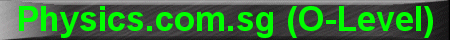(New tips are continually added to these pages.  Check back in a few months' time for more)

TOPIC 17:    Atomic Physics

Tip 1:

In radioactivity, the Half-Life T˝ is the expected time taken for half the radioactive nuclei to decay.

For example, if T˝ is 3 hours and we started off with only 32 radioactive nuclei.  After 3 hours, we will be left with 16; after another 3 hours (ie. total 6 hours), we will be left with 8; after yet another 3 hours (ie. total 9 hours), we will be left with 4 radioactive nuclei, and so on.

The general formula to compute the number of radioactive nuclei remaining is:

N = No (˝)n

where N is the number of radioactive nuclei remaining;  No the number initially; "n" the number of Half-Lives, ie. n = t/T˝ where t is the time that has elapsed.

Using the same example as above, when the time elapsed was 6 hours, then n = 6/3 = 2.

N = No (˝)n  = 32 (˝)2 = 32 (Ľ) = 8

This formula is especially useful if the time "t" is less than one Half-Life, eg. 2 hours.  Then, n = 2/3 = 0.667

N = No (˝)n  = 32 (˝)0.667 = 32 (0.63) = 20